经常听别人说这是一个看脸的世界，初听貌似很有道理，但细细一想却觉得也不尽然。君不见，那些实力不济的流量小花被大批粉丝追捧的同时，也一样会受到吃瓜群众的嘘声和嘲笑。那么，只看实力，外表就不重要吗？也不是。只有颜值与内涵并重，二者兼具，才能经受住时间的侵蚀，化为经典留在人们心中。在汽车界，就有这样一个才貌双全的美人儿，它就是红旗CA770。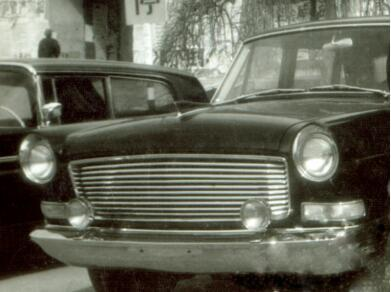在这个年轻人逐渐成为消费主力的时代，越来越多的产品都在探索富有运动感的设计。而在那个年代，对于汽车的设计各不相同，比如德国车，它的特点就是庄严，不管是奔驰、奥迪还是宝马，都是非常严肃的车型，因为它们经过了工业革命。英国车则显得比较绅士，比较保守。日本车一看就是那些小巧玲珑，比较讨人喜欢的东西较多。因此，作为红旗里最漂亮的车型，红旗CA770对动感的追求早已经走在时代的前列。从它整个造型来看，你就明白它为何能站住脚。只要你把它往任何地方一放，无论是正面还是侧面都相当有动感。
除强调动感外，CA770在融合现代文化与传统文化的道路上也进行了大胆尝试。整体线条追求简洁大方，线脚也更加清晰明朗，现代感非常强烈，此外还具有鲜明的民族特点，这些均从车的造型和内饰中体现了出来。不信你看，CA770车身造型的线型，就利用了明式家具的线脚，并结合了空气动力学的原理，车型更富有动感和整体感。车厢里采用了红木、树根的切片和牛皮、织锦缎等材料，体现了民族风格。
CA770的外形更加精致和协调，突破了CA72原来的“大、平、正、方”的效果。从前脸看，圆形的前大灯被保留了下来，原来扇形的水箱格栅被抽象化，作为设计元素，显得更合理。A柱的倾斜度增大，C柱到尾窗的设计也不再圆滑，表现得更加有力。由于积累了相当的经验，CA770的造型在表现中国传统风格的同时，又遵循了工业设计的原则，没有生硬地照搬，这种设计理念在今天也有很高的借鉴价值。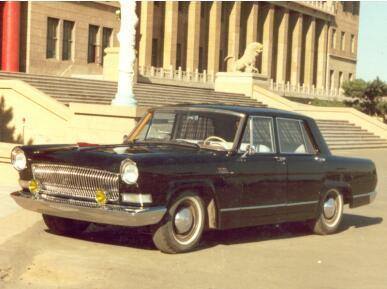说了这么多红旗CA770的高颜值，我们再来看看它的内在。很多人不知道，我国开发红旗CA770的时候，就已经做了风洞试验，这对当时的中国汽车工业来说是史无前例的。这就体现了一汽人的严谨科学的精神和迎难而上的气魄，没有条件制造条件也要上。他们经过仔细研究，决定在哈尔滨飞机厂做1:5风洞试验。通过在汽车模型中放许多线头，用风吹，看这些线头走向，从而了解汽车模型的风的阻力，确定红旗车如何进行合理修改。尽管风洞试验以现在的眼光来看比较简陋，但是也达到了空气动力学的测试要求。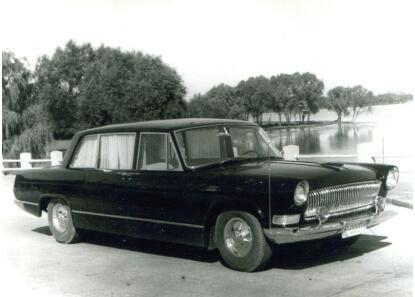1965年9月12日，凝结着一汽人智慧的CA770型三排座红旗牌高级轿车诞生了。这款车增加的新型升降隔音设备和后座可移动机构让人惊艳。当高级轿车送到北京后，在全国引起巨大轰动，首长们也对它非常喜爱。据说有的首长坐上新车后将时速开到了一百公里，却仍没有多少颠簸的感觉，并对红旗CA770的动力性能与稳定性赞叹不已。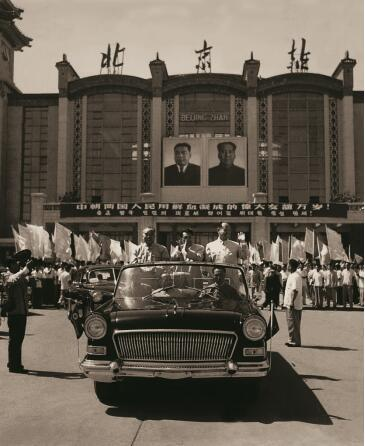作为红旗轿车的突破之作，红旗CA770难挡国人对它的喜爱之情。1966年4月20日，干劲冲天的一汽人经过五个多月的艰苦奋斗，克服重重困难，提前十天制造完成了20辆新型红旗三排座高级轿车，并立即送往北京。4月21日，新车运到北京永定门车站后，早有兴奋的群众等待，希望一睹这款车的盛世美颜。20辆新车由永定门车站沿着东西长安街行驶，两侧行人驻足观望，频频向司机举手致意，一汽送车的同志们都深为感动。这批车到京后，首长们竟带头为红旗车打起了广告，以后国家的任何重大活动都能看到红旗车的身影。
历史不会忘记，为民族品牌红旗轿车做出巨大贡献的一代汽车人；历史更不会忘记，那些一直关注并积极支持民族轿车工业的国人。 如今，新一代一汽人也像老一辈无产阶级革命家一样深爱着红旗，与红旗同欢乐、共命运，不论设计、生产、销售还是服务，不论前方将士还是辅助宣传单位，都日思夜想红旗，为豪华红旗品牌加班加点，殚精竭虑，愿为红旗插上一飞冲天的翅膀。并盼望着，待到山花烂漫时，红旗丛中笑！

##### 相关资讯

|分享

•已阅！握爪
•我手滑为你点赞
•128个赞！
•不明觉厉
•阅后既醉
•有钱！任性
•照片太美，我不敢看
•干货！杠杠的
•高大上
•膜拜中！
•请接受我的膝盖
•猫哥/猫妹么么哒
•神吐槽
•我只笑笑不说话
•我想静静
•窒息
##### 资讯相关车系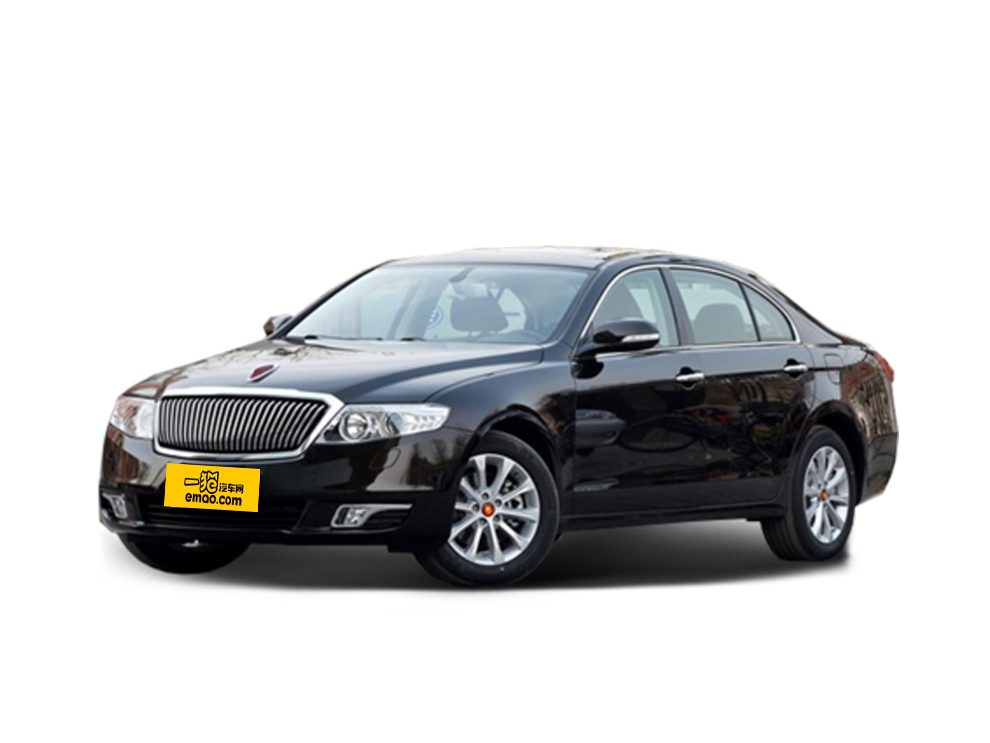##### 热度排行• 阿斯顿·马丁
• 奥迪
• 阿尔法罗密欧
• ALPINA

• 巴博斯
• 宝骏
• 宝马
• 保时捷
• 北汽制造
• 奔驰
• 奔腾
• 本田
• 比亚迪
• 标致
• 别克
• 宾利
• 布加迪
• 北汽威旺
• 北京
• 北汽绅宝
• 北汽幻速
• 北汽新能源
• 宝沃
• 比速汽车
• 北汽道达

• 昌河
• 长安
• 长城
• 长安商用
• 成功汽车
• 长江EV
• 长安轻车型

• 大众
• 道奇
• 东风
• 东风风神
• 东风小康
• 东南
• DS
• 东风风行
• 东风风度
• 东风风光

• 法拉利
• 菲亚特
• 丰田
• 福迪
• 福特
• 福田汽车
• 福汽启腾
• 风诺

• GMC
• 广汽传祺
• 广汽吉奥
• 观致

• 哈飞
• 海格
• 海马
• 华泰
• 黄海
• 恒天
• 红旗
• 哈弗
• 华颂
• 华凯
• 华泰新能源
• 汉腾汽车

• Jeep
• 江淮
• 江铃
• 捷豹
• 金杯
• 九龙
• 吉利汽车
• 金旅
• 金龙
• 江铃集团轻汽
• 江铃集团新能源
• 君马汽车
• 捷途

• 开瑞
• 凯迪拉克
• 科尼赛克
• 克莱斯勒
• KTM
• 卡威
• 凯翼
• 康迪
• 康迪电动汽车集团

• 猎豹汽车
• 兰博基尼
• 劳斯莱斯
• 雷克萨斯
• 雷诺
• 理念
• 力帆
• 莲花汽车
• 林肯
• 铃木
• 陆风
• 路虎
• 路特斯
• 领志
• 领克
• 零跑汽车

• MG
• MINI
• 马自达
• 玛莎拉蒂
• 迈凯伦
• 摩根

• 纳智捷

• 讴歌
• 欧宝
• 欧朗
• 欧拉
• 欧尚汽车

• 帕加尼

• 奇瑞
• 启辰
• 起亚
• 前途
• 庆铃汽车

• 日产
• 荣威
• 瑞麒汽车
• 如虎
• 瑞驰

• 上汽大通
• smart
• 三菱
• 双环
• 双龙
• 斯巴鲁
• 斯柯达
• 萨博
• 思铭
• 赛麟
• SWM斯威汽车

• TESLA
• 泰卡特
• 腾势

• 威麟
• 威兹曼
• 沃尔沃
• 五菱汽车
• 五十铃
• 潍柴英致
• WEY
• 蔚来
• 威马汽车

• 现代
• 雪佛兰
• 雪铁龙
• 西雅特
• 新特汽车
• 小鹏汽车
• 新宝骏

• 野马汽车
• 一汽
• 依维柯
• 英菲尼迪
• 永源
• 驭胜

• 中华
• 中兴
• 众泰
• 知豆
• 之诺
• 正道汽车
• A
• B
• C
• D
• E
• F
• G
• H
• I
• J
• K
• L
• M
• N
• O
• P
• Q
• R
• S
• T
• U
• V
• W
• X
• Y
• Z

• 阿斯顿·马丁
• 奥迪
• 阿尔法罗密欧
• ALPINA

• 巴博斯
• 宝骏
• 宝马
• 保时捷
• 北汽制造
• 奔驰
• 奔腾
• 本田
• 比亚迪
• 标致
• 别克
• 宾利
• 布加迪
• 北汽威旺
• 北京
• 北汽绅宝
• 北汽幻速
• 北汽新能源
• 宝沃
• 比速汽车
• 北汽道达

• 昌河
• 长安
• 长城
• 长安商用
• 成功汽车
• 长江EV
• 长安轻车型

• 大众
• 道奇
• 东风
• 东风风神
• 东风小康
• 东南
• DS
• 东风风行
• 东风风度
• 东风风光

• 法拉利
• 菲亚特
• 丰田
• 福迪
• 福特
• 福田汽车
• 福汽启腾
• 风诺

• GMC
• 广汽传祺
• 广汽吉奥
• 观致

• 哈飞
• 海格
• 海马
• 华泰
• 黄海
• 恒天
• 红旗
• 哈弗
• 华颂
• 华凯
• 华泰新能源
• 汉腾汽车

• Jeep
• 江淮
• 江铃
• 捷豹
• 金杯
• 九龙
• 吉利汽车
• 金旅
• 金龙
• 江铃集团轻汽
• 江铃集团新能源
• 君马汽车
• 捷途

• 开瑞
• 凯迪拉克
• 科尼赛克
• 克莱斯勒
• KTM
• 卡威
• 凯翼
• 康迪
• 康迪电动汽车集团

• 猎豹汽车
• 兰博基尼
• 劳斯莱斯
• 雷克萨斯
• 雷诺
• 理念
• 力帆
• 莲花汽车
• 林肯
• 铃木
• 陆风
• 路虎
• 路特斯
• 领志
• 领克
• 零跑汽车

• MG
• MINI
• 马自达
• 玛莎拉蒂
• 迈凯伦
• 摩根

• 纳智捷

• 讴歌
• 欧宝
• 欧朗
• 欧拉
• 欧尚汽车

• 帕加尼

• 奇瑞
• 启辰
• 起亚
• 前途
• 庆铃汽车

• 日产
• 荣威
• 瑞麒汽车
• 如虎
• 瑞驰

• 上汽大通
• smart
• 三菱
• 双环
• 双龙
• 斯巴鲁
• 斯柯达
• 萨博
• 思铭
• 赛麟
• SWM斯威汽车

• TESLA
• 泰卡特
• 腾势

• 威麟
• 威兹曼
• 沃尔沃
• 五菱汽车
• 五十铃
• 潍柴英致
• WEY
• 蔚来
• 威马汽车

• 现代
• 雪佛兰
• 雪铁龙
• 西雅特
• 新特汽车
• 小鹏汽车
• 新宝骏

• 野马汽车
• 一汽
• 依维柯
• 英菲尼迪
• 永源
• 驭胜

• 中华
• 中兴
• 众泰
• 知豆
• 之诺
• 正道汽车
• A
• B
• C
• D
• E
• F
• G
• H
• I
• J
• K
• L
• M
• N
• O
• P
• Q
• R
• S
• T
• U
• V
• W
• X
• Y
• Z

• 阿斯顿·马丁
• 奥迪
• 阿尔法罗密欧
• ALPINA

• 巴博斯
• 宝骏
• 宝马
• 保时捷
• 北汽制造
• 奔驰
• 奔腾
• 本田
• 比亚迪
• 标致
• 别克
• 宾利
• 布加迪
• 北汽威旺
• 北京
• 北汽绅宝
• 北汽幻速
• 北汽新能源
• 宝沃
• 比速汽车
• 北汽道达

• 昌河
• 长安
• 长城
• 长安商用
• 成功汽车
• 长江EV
• 长安轻车型

• 大众
• 道奇
• 东风
• 东风风神
• 东风小康
• 东南
• DS
• 东风风行
• 东风风度
• 东风风光

• 法拉利
• 菲亚特
• 丰田
• 福迪
• 福特
• 福田汽车
• 福汽启腾
• 风诺

• GMC
• 广汽传祺
• 广汽吉奥
• 观致

• 哈飞
• 海格
• 海马
• 华泰
• 黄海
• 恒天
• 红旗
• 哈弗
• 华颂
• 华凯
• 华泰新能源
• 汉腾汽车

• Jeep
• 江淮
• 江铃
• 捷豹
• 金杯
• 九龙
• 吉利汽车
• 金旅
• 金龙
• 江铃集团轻汽
• 江铃集团新能源
• 君马汽车
• 捷途

• 开瑞
• 凯迪拉克
• 科尼赛克
• 克莱斯勒
• KTM
• 卡威
• 凯翼
• 康迪
• 康迪电动汽车集团

• 猎豹汽车
• 兰博基尼
• 劳斯莱斯
• 雷克萨斯
• 雷诺
• 理念
• 力帆
• 莲花汽车
• 林肯
• 铃木
• 陆风
• 路虎
• 路特斯
• 领志
• 领克
• 零跑汽车

• MG
• MINI
• 马自达
• 玛莎拉蒂
• 迈凯伦
• 摩根

• 纳智捷

• 讴歌
• 欧宝
• 欧朗
• 欧拉
• 欧尚汽车

• 帕加尼

• 奇瑞
• 启辰
• 起亚
• 前途
• 庆铃汽车

• 日产
• 荣威
• 瑞麒汽车
• 如虎
• 瑞驰

• 上汽大通
• smart
• 三菱
• 双环
• 双龙
• 斯巴鲁
• 斯柯达
• 萨博
• 思铭
• 赛麟
• SWM斯威汽车

• TESLA
• 泰卡特
• 腾势

• 威麟
• 威兹曼
• 沃尔沃
• 五菱汽车
• 五十铃
• 潍柴英致
• WEY
• 蔚来
• 威马汽车

• 现代
• 雪佛兰
• 雪铁龙
• 西雅特
• 新特汽车
• 小鹏汽车
• 新宝骏

• 野马汽车
• 一汽
• 依维柯
• 英菲尼迪
• 永源
• 驭胜

• 中华
• 中兴
• 众泰
• 知豆
• 之诺
• 正道汽车
• A
• B
• C
• D
• E
• F
• G
• H
• I
• J
• K
• L
• M
• N
• O
• P
• Q
• R
• S
• T
• U
• V
• W
• X
• Y
• Z